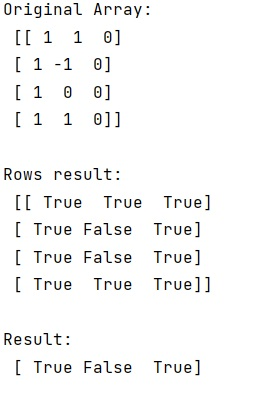# How to check if all values in the columns of a NumPy matrix are the same?

Learn, how to check if all values in the columns of a NumPy matrix are the same in Python?
Submitted by Pranit Sharma, on February 22, 2023

NumPy is an abbreviated form of Numerical Python. It is used for different types of scientific operations in python. Numpy is a vast library in python which is used for almost every kind of scientific or mathematical operation. It is itself an array which is a collection of various methods and functions for processing the arrays.

## Checking if all values in the columns of a NumPy matrix are the same

Suppose that we are given a NumPy array that mostly contains similar values, we need to check if all values in the columns of a numpy matrix are the same or not.

For this purpose, we can compare each value to the corresponding value in the first row and a column shares a common value if all the values in that column are True.

Let us understand with the help of an example,

## Python code to check if all values in the columns of a NumPy matrix are the same

```# Import numpy
import numpy as np

# Creating a numpy array
arr = np.array([[1,1,0],[1,-1,0],[1,0,0],[1,1,0]])

# Display original array
print("Original Array:\n",arr,"\n")

# Checking all rows
res = arr == arr[0,:]

# Display row result
print("Rows result:\n",res,"\n")

# Checking for columns
res = np.all(arr == arr[0,:], axis = 0)

# Display result
print("Result:\n",res,"\n")
```

Output: